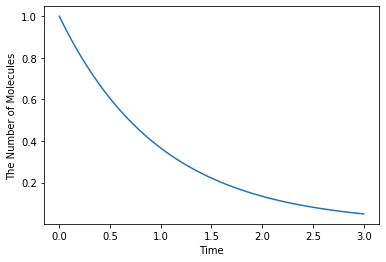# 6. How to Solve ODEs with Rate Law Functions¶

In general, ReactionRule describes a mass action kinetics with no more than two reactants. In case of a reaction with a complecated rate law, ReactionRule could be extensible with ReactionRuleDescriptor. Here, we explan the use of ReactionRuleDescriptor especially for ode.

:

%matplotlib inline
from ecell4.prelude import *


## 6.1. ReactionRuleDescriptor¶

ReactionRule defines reactants, products, and a kinetic rate.

:

rr1 = ReactionRule()
rr1.set_k(1.0)
print(len(rr1.reactants()))  # => 2
print(len(rr1.products()))  # => 1
print(rr1.k())  # => 1.0
print(rr1.as_string())  # => A+B>C|1
print(rr1.has_descriptor())  # => False

2
1
1.0
A+B>C|1
False


In addition to that, ReactionRule could be extensible with ReactionRuleDescriptor.

:

desc1 = ReactionRuleDescriptorMassAction(1.0)
print(desc1.k())
rr1.set_descriptor(desc1)

1.0


ReactionRuleDescriptor is accessible from ReactionRule.

:

print(rr1.has_descriptor())
print(rr1.get_descriptor())
print(rr1.get_descriptor().k())

True
<ecell4_base.core.ReactionRuleDescriptorMassAction object at 0x147e2c4765e0>
1.0


ReactionRuleDescriptor can store stoichiometric coefficients for each reactants:

:

desc1.set_reactant_coefficient(0, 2)  # Set a coefficient of the first reactant
desc1.set_reactant_coefficient(1, 3)  # Set a coefficient of the second reactant
desc1.set_product_coefficient(0, 4)  # Set a coefficient of the first product
print(rr1.as_string())

2*A+3*B>4*C|1


You can get the list of coefficients in the following way:

:

print(desc1.reactant_coefficients())  # => [2.0, 3.0]
print(desc1.product_coefficients())  # => [4.0]

[2.0, 3.0]
[4.0]


Please be careful that ReactionRuleDescriptor works properly only with ode.

## 6.2. ReactionRuleDescriptorPyFunc¶

ReactionRuleDescriptor provides a function to calculate a derivative (flux or velocity) based on the given values of Species. In this section, we will explain the way to define your own kinetic law.

:

rr1 = ReactionRule()
print(rr1.as_string())

A+B>C|0


First, define a rate law function as a Python function. The function must accept six arguments and return a floating number. The first and second lists contain a value for each reactants and products respectively. The third and fourth represent volume and time. The coefficients of reactants and products are given in the last two arguments.

:

def ratelaw(r, p, v, t, rc, pc):
return 1.0 * r * r - 2.0 * p


ReactionRuleDescriptorPyFunc accepts the function.

:

desc1 = ReactionRuleDescriptorPyfunc(ratelaw, 'test')
desc1.set_reactant_coefficients([1, 1])
desc1.set_product_coefficients()
rr1.set_descriptor(desc1)
print(desc1.as_string())
print(rr1.as_string())

test
1*A+1*B>1*C|0


A lambda function is available too.

:

desc2 = ReactionRuleDescriptorPyfunc(lambda r, p, v, t, rc, pc:  1.0 * r * r - 2.0 * p, 'test')
desc2.set_reactant_coefficients([1, 1])
desc2.set_product_coefficients()
rr1.set_descriptor(desc2)
print(desc1.as_string())
print(rr1.as_string())

test
1*A+1*B>1*C|0


To test if the function works properly, evaluate the value with ode.World.

:

w = ode.World()
w.set_value(Species("A"), 10)
w.set_value(Species("B"), 20)
w.set_value(Species("C"), 30)

print(w.evaluate(rr1))  # => 140 = 1 * 10 * 20 - 2 * 30

140.0


## 6.3. NetworkModel¶

NetworkModel accepts ReactionRules with and without ReactionRuleDescriptor.

:

m1 = NetworkModel()
rr1 = create_unbinding_reaction_rule(Species("C"), Species("A"), Species("B"), 3.0)
rr2 = create_binding_reaction_rule(Species("A"), Species("B"), Species("C"), 0.0)
desc1 = ReactionRuleDescriptorPyfunc(lambda r, p, v, t, rc, pc: 0.1 * r * r, "test")
desc1.set_reactant_coefficients([1, 1])
desc1.set_product_coefficients()
rr2.set_descriptor(desc1)


You can access to the list of ReactionRules in NetworkModel via its member reaction_rules().

:

print([rr.as_string() for rr in m1.reaction_rules()])

['C>A+B|3', '1*A+1*B>1*C|0']


Finally, you can run simulations in the same way with other solvers as follows:

:

run_simulation(1.0, model=m1, y0={'A': 60, 'B': 60})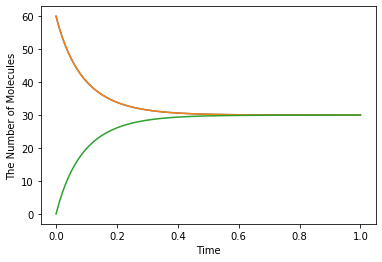Modeling with Python decorators is also available by specifying a function instead of a rate (floating number). When a floating number is set, it is assumed to be a kinetic rate of a mass action reaction, but not a constant velocity.

:

from functools import reduce
from operator import mul

with reaction_rules():
A + B == C | (lambda r, *args: 0.1 * reduce(mul, r), 3.0)

m1 = get_model()


For the simplicity, you can directory defining the equation with Species names as follows:

:

with reaction_rules():
A + B == C | (0.1 * A * B, 3.0)

m1 = get_model()


When you call a Species (in the rate law) which is not listed as a reactant or product, it is automatically added to the list as an enzyme.

:

with reaction_rules():
S > P | 1.0 * E * S / (30.0 + S)

m1 = get_model()
print(m1.reaction_rules().as_string())
print(m1.reaction_rules().get_descriptor().as_string())

1*S+1*E>1*P+1*E|0
((1.0 * E * S) / (30.0 + S))


where E in the equation is appended to both reacant and product lists.

:

run_simulation(10.0, model=m1, y0={'S': 60, 'E': 30})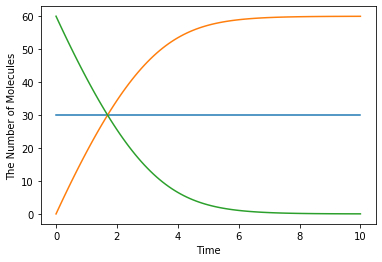Please be careful about typo in Species’ name. When you make a typo, it is unintentionally recognized as a new enzyme:

:

with reaction_rules():
A13P2G > A23P2G | 1500 * A13B2G  # typo: A13P2G -> A13B2G

m1 = get_model()
print(m1.reaction_rules().as_string())

1*A13P2G+1*A13B2G>1*A23P2G+1*A13B2G|0


When you want to disable the automatic declaration of enzymes, inactivate util.decorator.ENABLE_IMPLICIT_DECLARATION. If its value is False, the above case will raise an error:

:

import ecell4.util.decorator
ecell4.util.decorator.ENABLE_IMPLICIT_DECLARATION = False

try:
with reaction_rules():
A13P2G > A23P2G | 1500 * A13B2G
except RuntimeError as e:
print(repr(e))

ecell4.util.decorator.ENABLE_IMPLICIT_DECLARATION = True

RuntimeError('[A13B2G] is unknown [(1500 * {0})].')


Although E-Cell4 is specialized for a simulation of biochemical reaction network, by using a synthetic reaction rule, ordinary differential equations can be translated intuitively. For example, the Lotka-Volterra equations:

$\begin{split}\frac{dx}{dt} = Ax - Bxy\\\frac{dy}{dt} = -Cx + Dxy\end{split}$

where $$A=1.5, B=1, C=3, D=1, x(0)=10, y(0)=5$$, are solved as follows:

:

with reaction_rules():
A, B, C, D = 1.5, 1, 3, 1

~x > x | A * x - B * x * y
~y > y | -C * y + D * x * y

run_simulation(10, model=get_model(), y0={'x': 10, 'y': 5})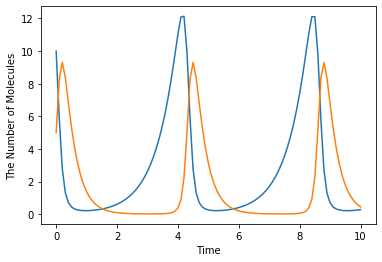## 6.4. References in a Rate Law¶

Here, we exlain the details in the rate law definition.

First, when you use simpler definitions of a rate law with Species, only a limited number of mathematical functions (e.g. exp, log, sin, cos, tan, asin, acos, atan, and pi) are available there even if you declare the function outside the block.

:

try:
from math import erf

with reaction_rules():
S > P | erf(S / 30.0)
except TypeError as e:
print(repr(e))

TypeError('must be real number, not DivExp')


This error happens because erf is tried to be evaluated agaist S / 30.0, which is not a floating number. In contrast, the following case is acceptable:

:

from math import erf

with reaction_rules():
S > P | erf(2.0) * S

m1 = get_model()
print(m1.reaction_rules().get_descriptor().as_string())

(0.9953222650189527 * S)


where only the result of erf(2.0), 0.995322265019, is passed to the rate law. Thus, the rate law above has no reference to the erf function. Similarly, a value of variables declared outside is acceptable, but not as a reference.

:

kcat, Km = 1.0, 30.0

with reaction_rules():
S > P | kcat * E * S / (Km + S)

m1 = get_model()
print(m1.reaction_rules().get_descriptor().as_string())
kcat = 2.0  # This doesn't affect the model
print(m1.reaction_rules().get_descriptor().as_string())

((1.0 * E * S) / (30.0 + S))
((1.0 * E * S) / (30.0 + S))


Even if you change the value of a variable, it does not affect the rate law.

On the other hand, when you use your own function to define a rate law, it can hold a reference to variables outside.

:

k1 = 1.0

with reaction_rules():
S > P | (lambda r, *args: k1 * r)  # referring k1

m1 = get_model()

ret1 = run_simulation(2, model=m1, y0={"S": 60})
k1 = 2.0  # This could change the result
ret2 = run_simulation(2, model=m1, y0={"S": 60})

plotting.plot_number_observer(ret1, '-', ret2, '--')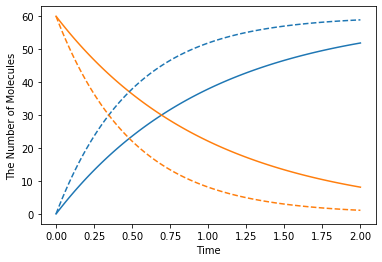However, in this case, it is better to make a new model for each set of parameters.

:

def create_model(k):
with reaction_rules():
S > P | k

return get_model()

ret1 = run_simulation(2, model=create_model(k=1.0), y0={"S": 60})
ret2 = run_simulation(2, model=create_model(k=2.0), y0={"S": 60})
plotting.plot_number_observer(ret1, '-', ret2, '--')In ode.World, a value for each Species is a floating number. However, for the compatibility, the common member num_molecules and add_molecules regard the value as an integer.

:

w = ode.World()
print(w.num_molecules(Species("A")))

2


To set/get a real number, use set_value and get_value:

:

w.set_value(Species("B"), 2.5)
print(w.get_value(Species("A")))
print(w.get_value(Species("B")))

2.5
2.5


As a default, ode.Simulator employs the Rosenblock method, called ROSENBROCK4_CONTROLLER, to solve ODEs. In addition to that, two solvers, EULER and RUNGE_KUTTA_CASH_KARP54, are available. ROSENBROCK4_CONTROLLER and RUNGE_KUTTA_CASH_KARP54 adaptively change the step size during time evolution due to error controll, but EULER does not.

:

with reaction_rules():
A > ~A | 1.0

m1 = get_model()

w1 = ode.World()
w1.set_value(Species("A"), 1.0)
sim1 = ode.Simulator(w1, m1, ode.EULER)
sim1.set_dt(0.01) # This is only effective for EULER
obs1 = FixedIntervalNumberObserver(0.1)
sim1.run(3.0, obs1)


ode.Factory also accepts a solver type and a default step interval.

:

run_simulation(3.0, model=m1, y0={"A": 1.0}, solver=('ode', ode.EULER, 0.01))